# 从C语言到C++⑩(第四章_模板初阶+STL简介)如何学习STL

1. 泛型编程

1.1 函数重载弊端

1.2 泛型编程概念

2. 函数模板

2.1 函数模板的概念

2.2  函数模板格式

2.3  函数模板原理

2.4 函数模板实例化

2.4.1 隐式实例化

2.4.2 显式实例化

2.5 模板参数的匹配原则

3. 类模板

3.1 类模板的定义

3.2 类模板实例化

4. 模板初阶笔试选择题

5. STL简介（了解）

5.1 什么是STL

5.2 STL的版本

5.3 STL的六大组件

5.4 如何学习STL

## 1. 泛型编程

### 1.1 函数重载弊端

#include<iostream>
using namespace std;

void Swap(int& left, int& right)
{
int temp = left;
left = right;
right = temp;
}
void Swap(double& left, double& right)
{
double temp = left;
left = right;
right = temp;
}
void Swap(char& left, char& right)
{
char temp = left;
left = right;
right = temp;
}

int main()
{
int a = 0, b = 1;
double c = 1.1, d = 2.2;
char e = 'e', f = 'f';

Swap(a, b);
Swap(c, d);
Swap(e, f);

return 0;
}

① 重载的函数仅仅是类型不同，代码复用率比较低，只要有新类型出现时，
就需要用户自己增加对应的函数
② 代码的可维护性比较低，一个出错可能所有的重载均出错

## 2. 函数模板

### 2.2  函数模板格式

template<typename T1, typename T2,......,typename Tn>

① template 是定义模板的关键字，后面跟的是尖括号 < >

也可以使用class(切记：不能使用struct代替class)

② typename 是用来定义模板参数的关键字

③ T1, T2, ..., Tn 表示的是函数名，可以理解为模板的名字，名字你可以自己取。

#include<iostream>
using namespace std;

template<typename T>
void Swap(T& left, T& right)
{
T temp = left;
left = right;
right = temp;
}

int main()
{
int a = 0, b = 1;
double c = 1.1, d = 2.2;
char e = 'e', f = 'f';

Swap(a, b);
Swap(c, d);
Swap(e, f);

cout << a << " " << b << endl;

return 0;
}

### 2.3  函数模板原理

#include<iostream>
using namespace std;

template<typename T>
void Swap(T& left, T& right)
{
T temp = left;
left = right;
right = temp;
}

int main()
{
int a = 0, b = 1;
double c = 1.1, d = 2.2;
char e = 'e', f = 'f';

Swap(a, b);
Swap(c, d);
Swap(e, f);

return 0;
}

（大小可能是1字节/4字节/8字节......）

### 2.4 函数模板实例化

int a = 0, b = 1;
Swap(a, b);

template<typename T>
void Swap(T& left, T& right)
{
T temp = left;
left = right;
right = temp;
}

#### 2.4.1 隐式实例化

#include <iostream>
using namespace std;

template<class T>
T Add(const T& x, const T& y)
{
return x + y;
}

int main()
{
int a1 = 10, a2 = 20;
double d1 = 10.1, d2 = 20.2;
cout << Add(a1, a2) << endl;
cout << Add(d1, d2) << endl;

return 0;
}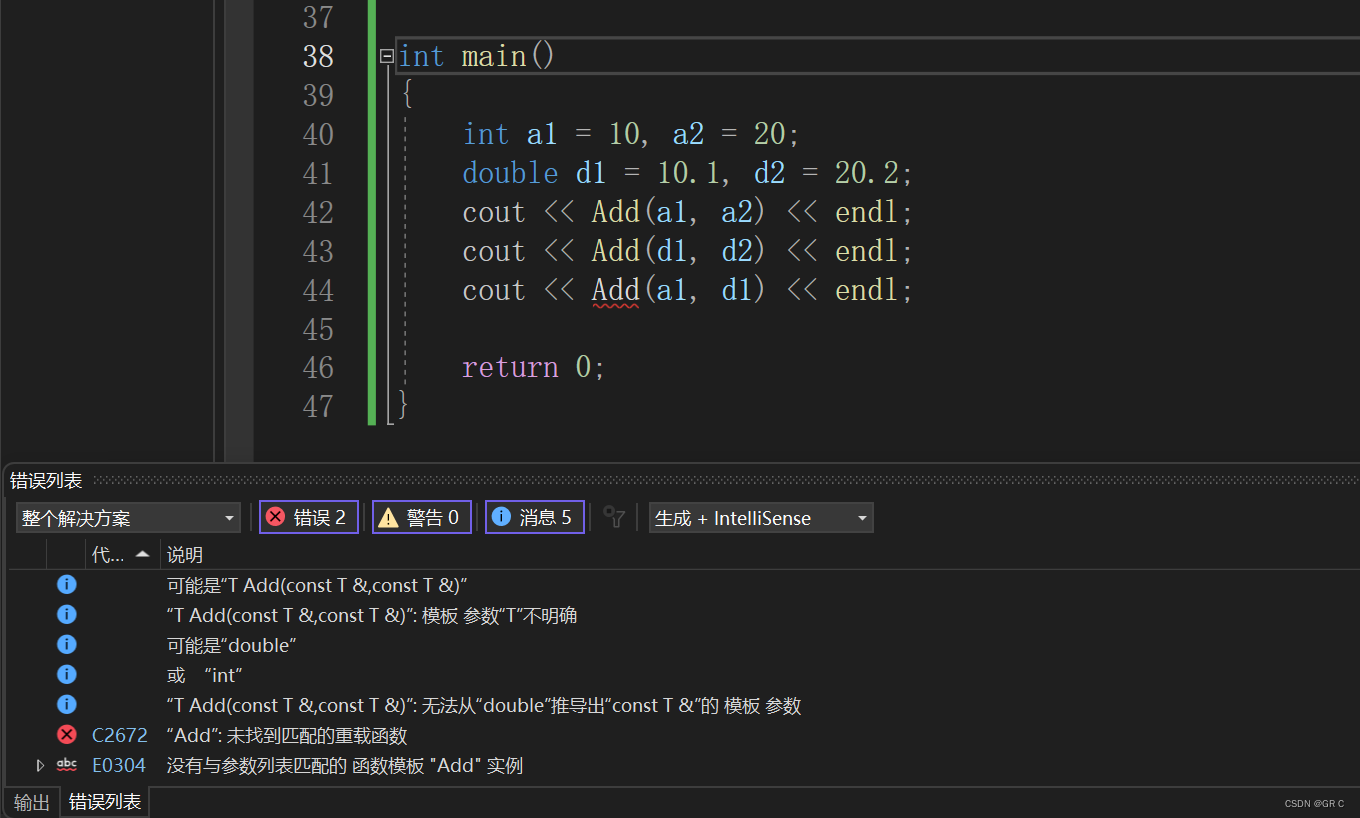这必然是失败的， 因为会出现冲突。编译器怎么知道T是什么呢？

① 传参之前先进行强制类型转换，非常霸道的解决方式：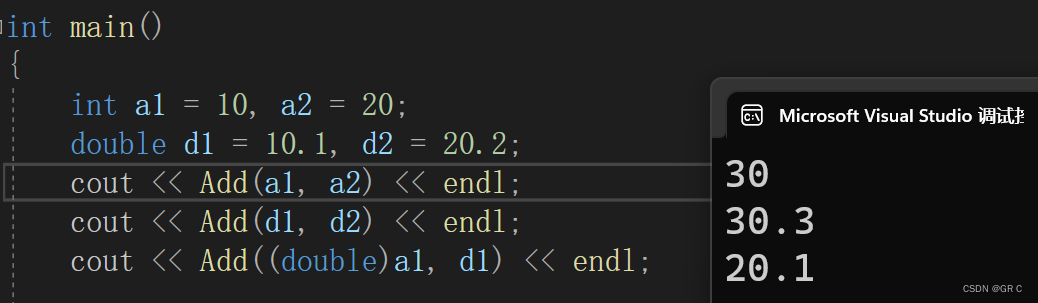② 写两个参数，那么返回的参数类型就会起决定性作用：

#include <iostream>
using namespace std;

template<class T1, class T2>
T1 Add(const T1& x, const T2& y) // 那么T1就是int，T2就是double
{
return x + y;      // 范围小的会像范围大的提升，int会像double "妥协"
} // 最后表达式会是一个double，但是最后返回值又是T1，是int，又会转

int main()
{
int a1 = 10, a2 = 20;
double d1 = 10.1, d2 = 20.2;

cout << Add(a1, d1) << endl;

return 0;
}

当然，这种问题严格意义上来说是不会用多个参数来解决的，

③ 我们还可以使用 "显式实例化" 来解决：

Add<int>(a1, d2);     // 指定实例化成int
Add<double>(a1, d2)   // 指定实例化成double

#### 2.4.2 显式实例化

（在函数名和参数列表中间加尖括号）

#include <iostream>
using namespace std;

template<class T>
T Add(const T& x, const T& y)
{
return x + y;
}

int main()
{
int a1 = 10, a2 = 20;
double d1 = 10.1, d2 = 20.2;
cout << Add(a1, a2) << endl;
cout << Add(d1, d2) << endl;

cout << Add<int>(a1, d2) << endl;     // 指定T用int类型
cout << Add<double>(a1, d2) << endl;  // 指定T用double类型

return 0;
}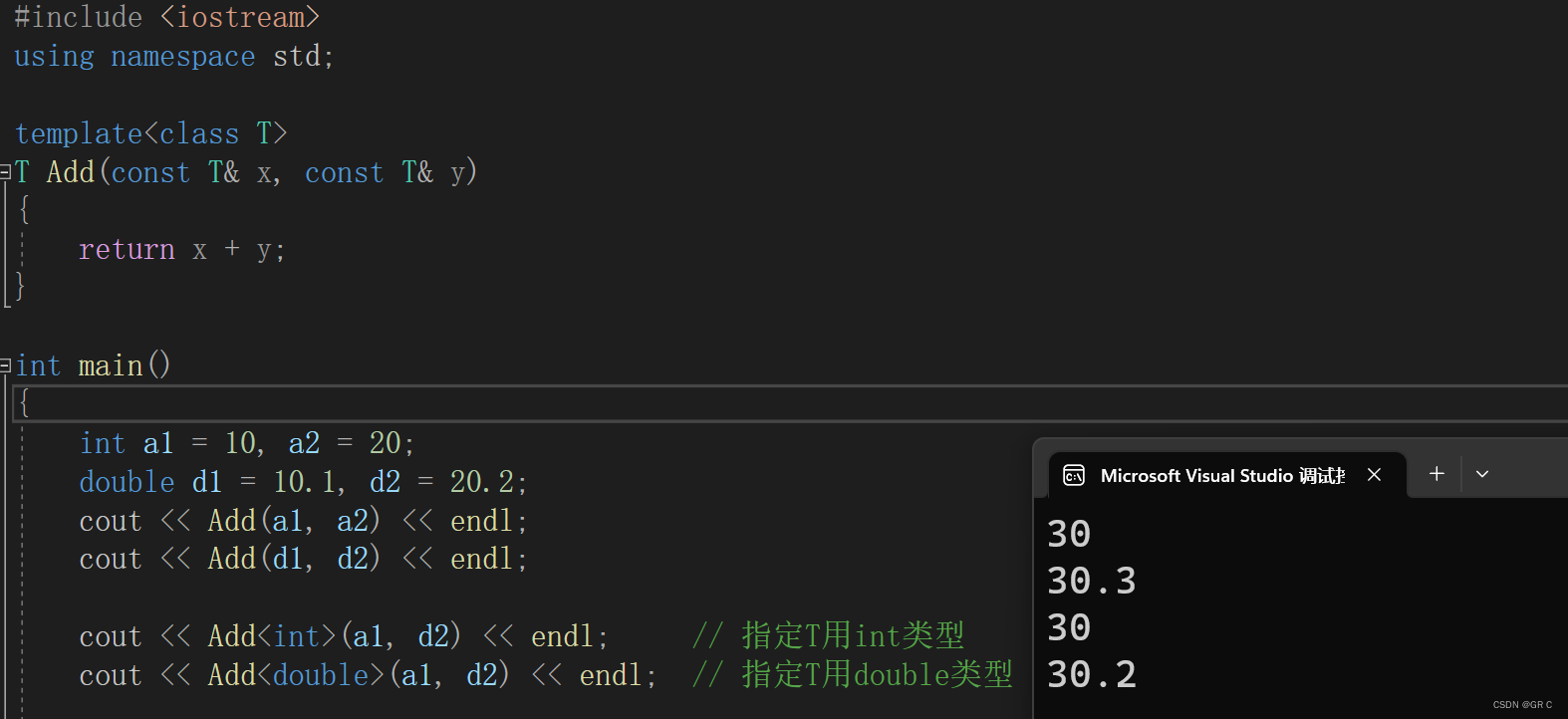第一个 Add<int>(a1, a2)  ，a2 是 double，它就要转换成 int 。

### 2.5 模板参数的匹配原则

#include <iostream>
using namespace std;

template<class T>
T Add(const T& x, const T& y)
{
cout << "T" << endl;
return x + y;
}

{
cout << "int" << endl;
return x + y;
}

int main()
{
int a1 = 10, a2 = 20;
cout << Add(a1, a2) << endl;

return 0;
}

匹配原则：

① 一个非模板函数可以和一个同名的模板函数同时存在，

#include <iostream>
using namespace std;

template<class T>
T Add(const T& x, const T& y)
{
cout << "T" << endl;
return x + y;
}

{
cout << "int" << endl;
return x + y;
}

int main()
{
int a1 = 10, a2 = 20;

return 0;
}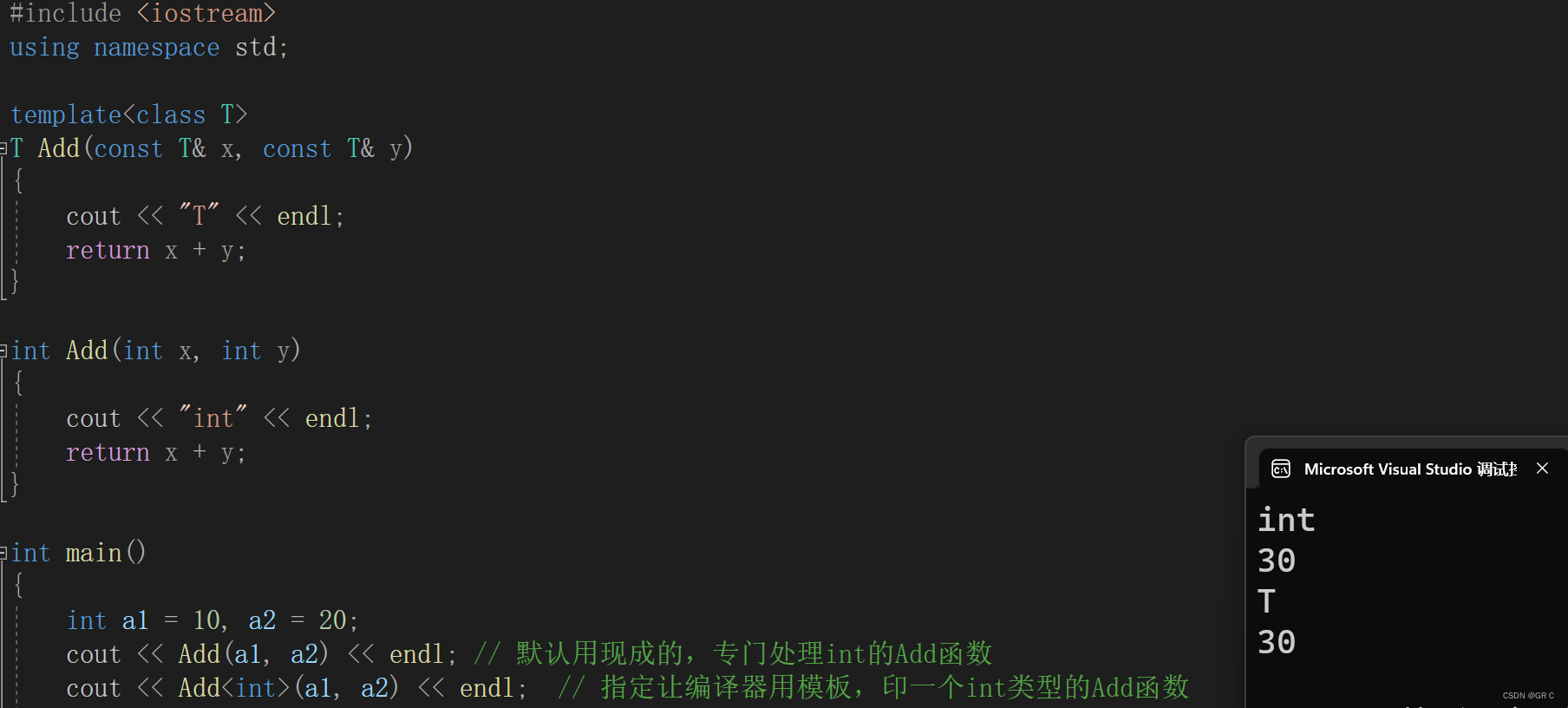② 对于非模板函数和同名函数模板，如果其他条件都相同，

#include <iostream>
using namespace std;

template<class T1, class T2>
T1 Add(const T1& x, const T2& y)
{
cout << "T" << endl;
return x + y;
}

{
cout << "int" << endl;
return x + y;
}

int main()
{
cout << Add(1, 2) << endl;     // 用现成的
//（与非函数模板类型完全匹配，不需要函数模板实例化）

cout << Add(1, 2.0) << endl;   // 可以，但不是很合适，自己印更好

return 0;
}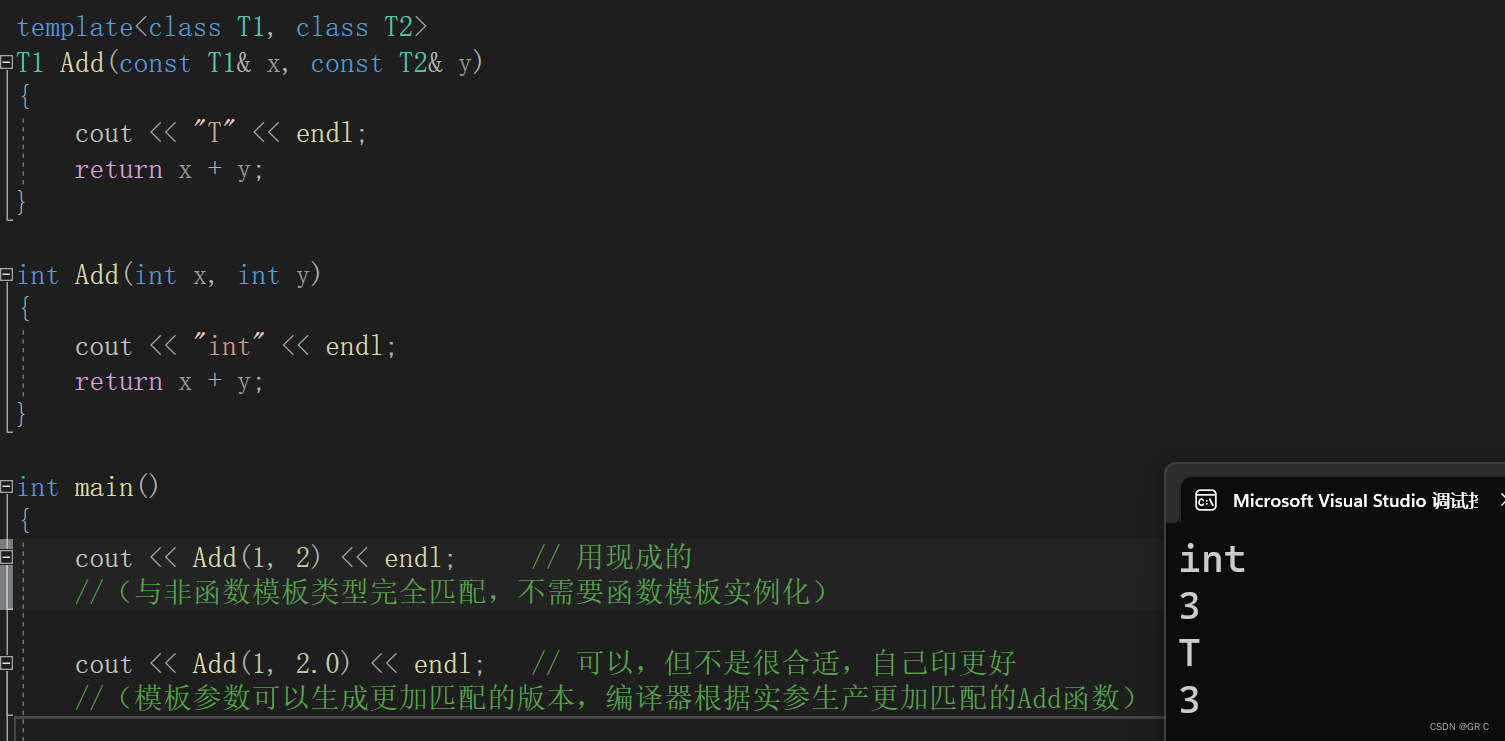## 3. 类模板

C语言在讲数据结构的时候，要转化存的类型，是用 typedef 来解决的。

int main()
{
Stack st1;   // 存int数据
Stack st2;   // 存double数据

return 0;
}

### 3.1 类模板的定义

template<class T1, class T2, ..., class Tn>
class 类模板名
{
// 类内成员定义
};

template<class T>
class Stack
{
public:
Stack(T capacity = 4)
: _top(0)
, _capacity(capacity)
{
_arr = new T[capacity];
}

~Stack();// 让析构函数放在类外定义
private:
T* _arr;
int _top;
int _capacity;
};

// 类模板中函数放在类外进行定义时，需要加模板参数列表
template <class T>
Stack<T>::~Stack()
{
delete[] _arr;
_arr = nullptr;
_capacity = _top = 0;
}

### 3.2 类模板实例化

#include <iostream>
using namespace std;

template<class T>
class Stack
{
public:
Stack(T capacity = 4)
: _top(0)
, _capacity(capacity)
{
_arr = new T[capacity];
}
~Stack()
{
delete[] _arr;
_arr = nullptr;
_capacity = _top = 0;
}
private:
T* _arr;
int _top;
int _capacity;
};

int main()
{
Stack<int> st1;   // 存储int
Stack<double> st2;   // 存储double

return 0;
}

① Stack 不是具体的类，是编译器根据被实例化的类型生成具体类的模具。

template<class T>
class Stack {...};

② Stack 是类名，Stack<int> 才是类型：

Stack<int> s1;
Stack<double> s2;

## 4. 模板初阶笔试选择题

4.1 下面有关C++中为什么用模板类的原因，描述错误的是? ( ）

A.可用来创建动态增长和减小的数据结构

B.它是类型无关的，因此具有很高的可复用性

C.它运行时检查数据类型，保证了类型安全

D.它是平台无关的，可移植性

4.2 在下列对fun的调用中，错误的是（ ）

﻿template <class T>
T fun(T x,T y)
{
return x*x+y*y;
}

A.fun(1, 2)

B.fun(1.0, 2)

C.fun(2.0, 1.0)

D.fun<float>(1, 2.0)

4.3 下列关于模板的说法正确的是（ ）

A.模板的实参在任何时候都可以省略

B.类模板与模板类所指的是同一概念

C.类模板的参数必须是虚拟类型的

D.类模板中的成员函数全是模板函数

4.4 下列的模板声明中，其中几个是正确的（ ）

﻿1)template

2)template<T1,T2>

3)template<class T1,T2>

4)template<class T1,class T2>

5)template<typename T1,T2>

6)template<typename T1,typename T2>

7)template<class T1,typename T2>

8)<typename T1,class T2>

A.2

B.3

C.4

D.5

4.5 下列描述错误的是（ ）

A.编写与类型无关的通用代码，是代码复用的一种手段。模板是泛型编程的基础

B.函数模板是一个蓝图，它本身并不是函数，是编译器用使用方式产生特定具体类型函数的模具

C.模板分为函数模板和类模板

D. 模板类跟普通类以一样的，编译器对它的处理时一样的

4.1 C

A.模板可以具有非类型参数，用于指定大小，可以根据指定的大小创建动态结构

B.模板最重要的一点就是类型无关，提高了代码复用性

C.模板运行时不检查数据类型，也不保证类型安全，相当于类型的宏替换，故错误

D.只要支持模板语法，模板的代码就是可移植的

4.2 B

A.通过参数推导，T为int,不存在二义性，调用正确

B.由于参数类型不一样，模板不支持类型转换，推导参数会产生二义性，编译错误

C.通过参数推导，T为float，不存在二义性，调用正确

D.通过类型实例化函数，调用正确

4.3 D

A.不一定，参数类型不同时有时需要显示指定类型参数

B.类模板是一个类家族，模板类是通过类模板实例化的具体类

C.C++中类模板的声明格式为template＜模板形参表声明＞＜类声明＞，

D.正确，定义时都必须通过完整的模板语法进行定义

4.4 B

A.1.模板语法错误，2.没有关键字class或typename指定类型，3.T2缺少class或typename

B.正确， 4,6,7为正确声明

C.5.T2缺少class或typename

D.8.缺少template

4.5 D

A.模板是代码复用的重要手段

B.函数模板不是一个具体函数，而是一个函数家族

C.目前涉及到的模板就两类，函数模板与类模板

D.模板类是一个家族，编译器的处理会分别进行两次编译，其处理过程跟普通类不一样

## 5. STL简介（了解）

### 5.1 什么是STL

STL —— Standard Template Libary——标准模板库，是 C++ 标准库的重要组成部分，

### 5.2 STL的版本

Alexander StepanovMeng Lee 惠普实验室完成的原始版本，本着开源精神，他们声明允许任何人任意运用、拷贝、修改、传播、商业使用这些代码，无需付费。唯一的条件就是也需要向原始版本一样做开源使用。 HP 版本--所有STL实现版本的始祖。

P. J. 版本

P. J. Plauger开发，继承自HP版本，被Windows Visual C++采用，不能公开或修改，

RW版本

Rouge Wage公司开发，继承自HP版本，被C+ + Builder 采用，不能公开或修改，可读性一般。

SGI版本

Silicon Graphics Computer SystemsInc公司开发，继承自HP版 本。

GCC(Linux)采用，可移植性好，

### 5.3 STL的六大组件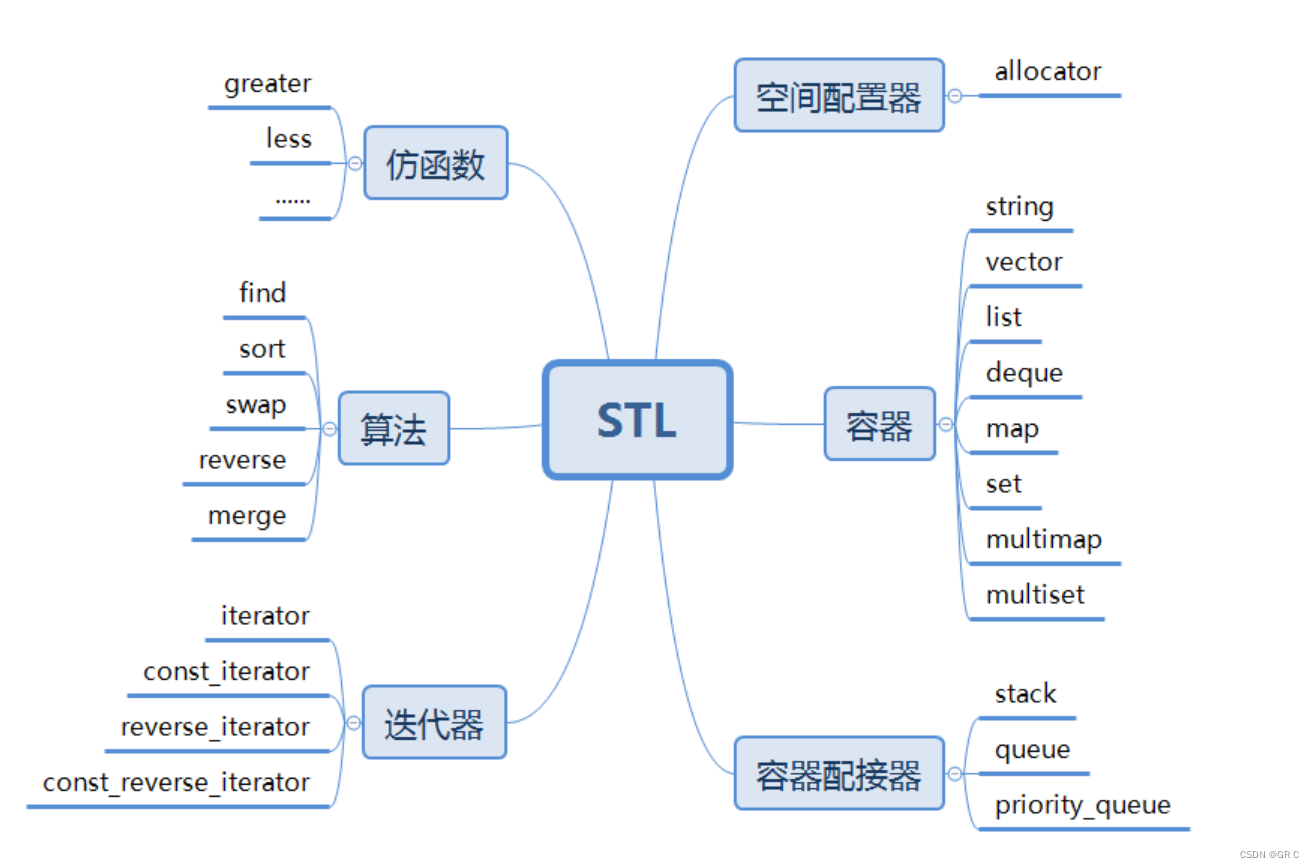STL提供六大组件，它们之间可以相互组合使用。

① 容器（containers）

② 算法（algorithms）

③ 迭代器（iterators）

④ 仿函数（functors）

⑥ 配置器（allocators）

### 5.4如何学习STL

C++刷题基本都会用到STL，STL C++ 中的优秀作品，有STL后，许多底层的数据结构

《Effctive C++》《高质量C++》《STL源码剖析》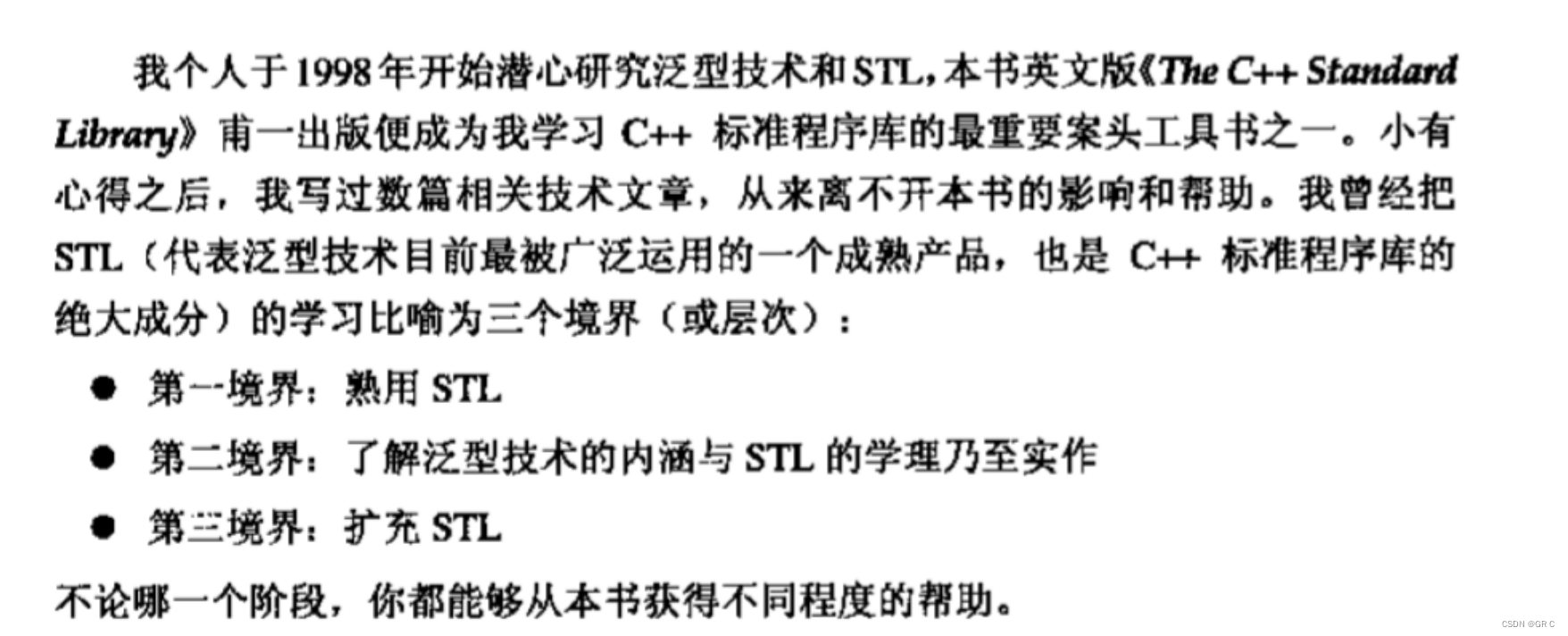本专栏后面就马上更新STL的内容了，学完消耗了应该就到第二境界了。

## 本章完。

09-15166
09-2295
09-2163
09-2242
09-15203
09-14274
09-1786
09-17190
09-16438
09-14504
09-2225
09-16285
09-16244
09-15331

### “相关推荐”对你有帮助么？

•非常没帮助
•没帮助
•一般
•有帮助
•非常有帮助被折叠的  条评论 为什么被折叠?到【灌水乐园】发言GR_C

¥1 ¥2 ¥4 ¥6 ¥10 ¥20获取中扫码支付点击重新获取扫码支付1.余额是钱包充值的虚拟货币，按照1:1的比例进行支付金额的抵扣。
2.余额无法直接购买下载，可以购买VIP、付费专栏及课程。余额充值